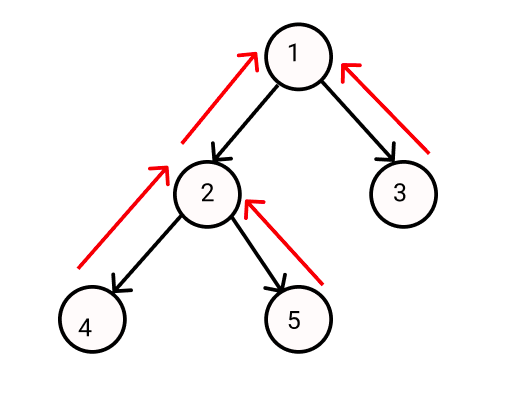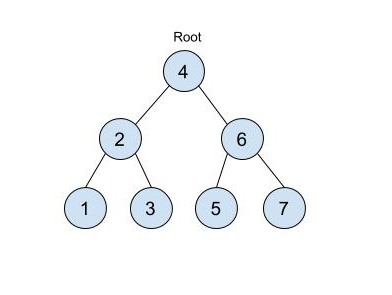New update is available. Click here to update.

# Ninja And Covid-19 Vaccine

Last Updated: 3 Mar, 2021
Difficulty: Moderate

## PROBLEM STATEMENT

#### Note :

``````The last house is similar to a leaf node in a binary tree and a house that is present at ‘K’ distance from the last house should be the direct ancestor of this last house.
``````

#### For Example :``````In this example :
2 is the direct ancestor of 4 and 5.
1 is the direct ancestor of 2, 3, 4, and 5.
``````
##### Input Format :
``````The first line of input contains an integer 'T' representing the number of test cases. Then the test cases follow.

The next line of each test case contains elements in the level order form. The line consists of values of nodes separated by a single space. In case a node is null, we take -1 on its place.

The next line of each test case contains one integer ‘K’ representing that the houses that are still not vaccinated are at a ‘K’ distance from the last houses in the city.

For example, the input for the tree depicted in the below image would be :
````````````4
2 6
1 3 5 7
-1 -1 -1 -1 -1 -1
``````

#### Explanation :

``````Level 1 :
The root node of the tree is 4

Level 2 :
Left child of 4 = 2
Right child of 4 = 6

Level 3 :
Left child of 2 = 1
Right child of 2 = 3
Left child of 6 = 5
Right child of 6 = 7

Level 4 :
Left child of 1 = null (-1)
Right child of 1 = null (-1)
Left child of 3 = null (-1)
Right child of 3 = null (-1)
Left child of 5 = null (-1)
Right child of 5 = null (-1)
Left child of 7 = null (-1)
Right child of 7 = null (-1)

The first not-null node(of the previous level) is treated as the parent of the first two nodes of the current level. The second not-null node (of the previous level) is treated as the parent node for the next two nodes of the current level and so on.
The input ends when all nodes at the last level are null(-1).
``````
##### Note :
``````The above format was just to provide clarity on how the input is formed for a given tree.

The sequence will be put together in a single line separated by a single space. Hence, for the above-depicted tree, the input will be given as:

4 2 6 1 3 5 7 -1 -1 -1 -1 -1 -1
``````
##### Output Format :
``````For each test case, print how many houses are there that are not vaccinated.

Print the output of each test case in a separate line.
``````
##### Constraints :
``````1 <= T <= 10
1 <= N <= 10000
1 <= data <= 100000

Where ‘N’ denotes the number of houses in the city and ‘data’ represents the number of people in each house.

Time Limit: 1 sec
``````

#### Note :

``````You do not need to print anything; it has already been taken care of. Just implement the given function.
``````